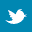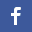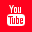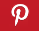Completely Solved C, C++ Programs Assignment.Quick Search Database Videos Tutorials Ebooks Forums FAQ Aboutus Household Industrial Manufacturing Service Shopping Transportation### C program to implement Breadth First Search and Depth First Search graph searching algorithms

 Filed Under: C Assignments

Program Statement :
Write a menu-driven C program to implement Breadth First Search and Depth First Search graph searching algorithms which will accept an input graph and will search any node given as input.

Theory :
Nodes of a graph can store data. For such a given graph we have to find presence of a node with a specified data element. To do so, we have to traverse the nodes of that graph. It is called graph searching. The final answer will be either present or absent after graph searching. The order of visiting the nodes may be different for different graph searching algorithms.
Depth First Search(DFS) searches a graph vertically or depth wise, thus going deeper and deeper in a graph until the target node is found or until a dead end (node that has no children) is found. Then the search backtracks, returning to the most recent node it hasn't finished exploring.
On the other hand, Breadth First Search(BFS) searches a graph horizontally or breadth wise. It exhaustively searches the entire graph until the target node is found or until a dead end is found.
To implement these algorithms, a graph is taken as input in the form of a vertex by vertex binary matrix such that
6. w(i,j) = 0 if vertices vi and vj has no path between them and
7. w(i,j) = 1 if there is a path from vi to vj.
We also need a status[] array which keeps track of the state of the vertices.
● status[i] = ready denotes ith vertex or node is not yet visited.
● status[i]=visited denotes ith vertex or node is already visited.
In both these algorithms, we consider a list named OPEN, which contains promising nodes to be expanded in future. If we implement OPEN as a queue data structure then the following algorithm is B.F.S and when OPEN is a stack then it is D.F.S.Algorithm :
/* The input graph, number of vertices and the target node are passed as parameters */

Algo_graph_search(a[max][max],v,targrt)
{
found=0; /*If search will be successful then value of found will be changed to 1*/
For(i=1 to v)
s = starting node; /*Searching starts from the first node of the graph*/
If(s=target) /*Check whether starting node is our target*/
{
found = 1;
return found;
}
Insert s in OPEN; /* OPEN is queue for B.F.S and stack for D.F.S */
While(OPEN is not empty)/* Searching will be considered until open is empty */
{
/*Deletion from open will follow deletion from queue for B.F.S and stack for D.F.S*/ t = Delete a node from open and store it in t;
status[t] = visited; /* The state of the deleted node becomes visited */
For(j=1 to v)
{
step1: Expand t by generating all of its successors tj;
step2: If (any tj is the target node)
{
found = 1;
return found;
}
step3: Insert only that tj s in OPEN which are not already visited
}
}
return found;
}
/* End of Algo_graph_search */

Program listing :
/ *C program to implement B.F.S and D.F.S for any graph */

#include<stdio.h>
#include<stdlib.h>
#define max 10/*Defining maximum number of vertices of the input graph*/
enum{True,False};
main()
{
int a[max][max],v,i,j,g,result,ch;
char chr;
int bfs(int[][j],int,int);
int dfs(int[][j],int,int);
printf("Enter the number of vertex : ");
scanf("%d",&v);
for(i=0;i<v;i++)
{
for(j=0;j<v;j++)
{
printf("Enter the value of a[%d][%d] : ",i,j);
scanf("%d",&a[i][j]);
}
}
printf("The matrix is :-n");
for(i=0;i<v;i++)
{
for(j=0;j<v;j++)
{
printf("%dt",a[i][j]);
}
printf("n");
}
while(1)
{
printf("Enter the node you want to search : ");
printf("nEnter choice : ");
scanf("%d",&ch);
switch(ch)
{
case 1:
{
printf("Traversed path for B.F.S : ");
result=bfs(a,v,g);
break;
}
case 2:
{
printf("Traversed path for D.F.S : ");
result=dfs(a,v,g);
break;
}
default:
{
printf("!!!!!Error!!!!!n!!!!!Invalid choice given!!!!!");
return;
}
}
if(result==1)/*result stores the output of graph searching i.e. successful or unsuccessful*/
printf("nSearch successful,target node is found.n");
else
printf("nSearch unsuccessful, entire graph is traversed but target node is not found.n");
printf("nWant to continue?(give 1 for yes) : ");/*The program will continue until the user wants to exit*/
scanf("%d",&chr);
if(chr!=1)
{
printf("The program will exit now.");
return;
}
}
}
/*The input graph,number of vertices and the target node are passed as parameters*/
int bfs(int a[max][max],int v,int g)
{
int s,i,t,j,open[max],status[max],f;
void insert(int[],int,int*);
int delete(int[],int*);
int queue_empty(int*,int*);
f=0;/*If search will be successful then value of f will be changed to 1*/
int front=0;
int rear=0;
for(i=0;i<v;i++)
s=0;/*Searchig starts from the first node of the graph*/
if(s==g)/*Check whether starting node is our target*/
{
printf("v%d",s);
f=1;
return f;
}
insert(open,s,&rear);/*Insert the starting node in the queue*/
while((queue_empty(&front,&rear))==False)/*Searching will be considered until queue is empty*/
{
t=delete(open,&front);/*t contains the node just deleted from the queue*/
printf("v%d->",t);
status[t]=visited;/*The state of the deleted node becomes visited*/
for(j=0;j<v;j++)
{
if(a[t][j]==1)
{
if(j==g)/*Checking whether any successor node of t is our target*/
{
printf("v%d",j);
f=1;
return f;
}
if(status[j]!=visited)
{
insert(open,j,&rear);/*Insert only that successors of t which are not already
visited*/
status[j]=visited;/*State of all successors become visited*/
}
}
}

}
printf("b bb ");
return f;
}
void insert(int queue[],int data,int* rear)
{
if(*rear>=max)
{
printf("Error overflown");
return;
}
queue[*rear]=data;
(*rear)++;
}
int delete(int queue[],int* front)
{
int data;
data=queue[*front];
(*front)++;
return data;
}

int queue_empty(int* front,int* rear)
{
if(*front==*rear)
return True;
else
return False;
}
/*The input graph,number of vertices and the target node are passed as parameters*/
int dfs(int a[max][max],int v,int g)
{
int s,i,t,j,open[max],status[max],f;
void push(int[],int,int*);
int pop(int[],int*);
int stack_empty(int*);
f=0;/*If search will be successful then value of f will be changed to 1*/
int top=0;
for(i=0;i<v;i++)
s=0;/*Searchig starts from the first node of the graph*/
if(s==g)/*Check whether starting node is our target*/
{
printf("v%d",s);
f=1;
return f;
}
push(open,s,&top);/*Insert the starting node in the stack*/
while(stack_empty(&top)==False)/*Searching will be considered until stack is empty*/
{
t=pop(open,&top);/*t contains the node just deleted from the stack*/
printf("v%d->",t);
status[t]=visited;/*The state of the deleted node becomes visited*/
for(j=0;j<v;j++)
{
if(a[t][j]==1)
{
if(j==g)/*Checking whether any successor node of t is our target*/
{
printf("v%d",j);
f=1;
return f;
}
if(status[j]!=visited)
{
push(open,j,&top);/*Insert only that successors of t which are not already visited*/
status[j]=visited;/*State of all successors become visited*/
}
}
}
}
printf("b bb ");
return f;
}
void push(int stack[],int data,int* top)
{
if(*top>=max)
{
printf("Error overflown");
return;
}
stack[*top]=data;
(*top)++;
}
int pop(int stack[],int *top)
{
int data;
(*top)--;
data=stack[*top];
return data;
}
int stack_empty(int *top)
{
if(*top==0)
return True;
else
return False;
}

Output :
Enter the number of vertex : 6
Enter the value of a : 0
Enter the value of a : 1
Enter the value of a : 1
Enter the value of a : 0
Enter the value of a : 0
Enter the value of a : 0
Enter the value of a : 0
Enter the value of a : 0
Enter the value of a : 0
Enter the value of a : 1
Enter the value of a : 1
Enter the value of a : 0
Enter the value of a : 0
Enter the value of a : 1
Enter the value of a : 0
Enter the value of a : 0
Enter the value of a : 1
Enter the value of a : 0
Enter the value of a : 0
Enter the value of a : 0
Enter the value of a : 0
Enter the value of a : 0
Enter the value of a : 1
Enter the value of a : 0
Enter the value of a : 0
Enter the value of a : 0
Enter the value of a : 0
Enter the value of a : 0
Enter the value of a : 0
Enter the value of a : 1
Enter the value of a : 0
Enter the value of a : 0
Enter the value of a : 0
Enter the value of a : 0
Enter the value of a : 0
Enter the value of a : 0

The matrix is :-
0 1 1 0 0 0
0 0 0 1 1 0
0 1 0 0 1 0
0 0 0 0 1 0
0 0 0 0 0 1
0 0 0 0 0 0

Enter the node you want to search : 4

2>.....Depth First Search.....

Enter choice : 1

Traversed path for B.F.S : v0->v1->v4
Search successful,target node is found.

Want to continue?(give 1 for yes) : 1

Enter the node you want to search : 4

2>.....Depth First Search.....

Enter choice : 2

Traversed path for D.F.S : v0->v2->v4
Search successful,target node is found.

Want to continue?(give 1 for yes) : 1

Enter the node you want to search : 7

2>.....Depth First Search.....

Enter choice : 1

Traversed path for B.F.S : v0->v1->v2->v3->v4->v5
Search unsuccessful, entire graph is traversed but target node is not found.

Want to continue?(give 1 for yes) : 1

Enter the node you want to search : 8

2>.....Depth First Search.....

Enter choice : 2

Traversed path for D.F.S : v0->v2->v4->v5->v1->v3
Search unsuccessful, entire graph is traversed but target node is not found.

Want to continue?(give 1 for yes) : 0
The program will exit now.

Discussions :
 SPACE COMPLEXITY :
Since all of the nodes of a level must be saved until their child nodes in the next level have been generated, the space complexity is proportional to the number of nodes at the deepest level. In the worst case the graph has a depth of 1 and all vertices must be stored, since it is exponential in the depth of the graph.
 TIME COMPLEXITY :
The worst case time complexity of both these algorithms is
O(| V | + | E |) = O(max(| V | , | E |)), [where | V | is the number of vertices and | E | is the number of edges of the graph] since every vertex and every edge will be explored in the worst case.
 Any one of the algorithms can be used to detect existence of a circuit for any graph given as input.
 These algorithms can be implemented both for directed as well as for undirected graphs.
 It should be noted that we must use a stack for D.F.S and a queue for B.F.S.
 Breadth-first search is complete. This means that if there is a solution, breadth-first search will find it regardless of the kind of graph. However, if the graph is infinite and there is no solution breadth-first search will diverge.
 For unit-step cost, breadth-first search is optimal. In general breadth-first search is not optimal since it always returns the result with the fewest edges between the starting node and the goal node.Back to main directory:Software Practical